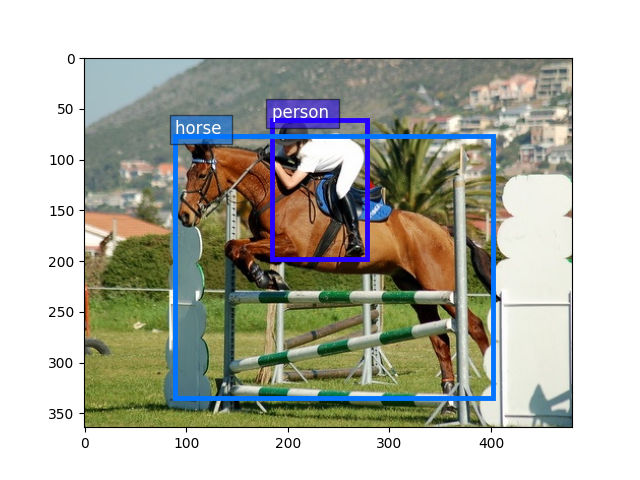# Prepare PASCAL VOC datasets¶

Pascal VOC is a collection of datasets for object detection. The most commonly combination for benchmarking is using 2007 trainval and 2012 trainval for training and 2007 test for validation. This tutorial will walk through the steps of preparing this dataset for GluonCV.Hint

You need 8.4 GB disk space to download and extract this dataset. SSD is preferred over HDD because of its better performance.

The total time to prepare the dataset depends on your Internet speed and disk performance. For example, it often takes 10 min on AWS EC2 with EBS.

## Prepare the dataset¶

We need the following four files from Pascal VOC:

Filename

Size

SHA-1

VOCtrainval_06-Nov-2007.tar

439 MB

34ed68851bce2a36e2a223fa52c661d592c66b3c

VOCtest_06-Nov-2007.tar

430 MB

41a8d6e12baa5ab18ee7f8f8029b9e11805b4ef1

VOCtrainval_11-May-2012.tar

1.9 GB

4e443f8a2eca6b1dac8a6c57641b67dd40621a49

benchmark.tgz

1.4 GB

7129e0a480c2d6afb02b517bb18ac54283bfaa35

The easiest way to download and unpack these files is to download helper script `pascal_voc.py` and run the following command:

```python pascal_voc.py
```

which will automatically download and extract the data into `~/.mxnet/datasets/voc`.

If you already have the above files sitting on your disk, you can set `--download-dir` to point to them. For example, assuming the files are saved in `~/VOCdevkit/`, you can run:

```python pascal_voc.py --download-dir ~/VOCdevkit
```

Loading images and labels is straight-forward with `gluoncv.data.VOCDetection`.

```from gluoncv import data, utils
from matplotlib import pyplot as plt

train_dataset = data.VOCDetection(splits=[(2007, 'trainval'), (2012, 'trainval')])
val_dataset = data.VOCDetection(splits=[(2007, 'test')])
print('Num of training images:', len(train_dataset))
print('Num of validation images:', len(val_dataset))
```

Out:

```Num of training images: 16551
Num of validation images: 4952
```

Now let’s visualize one example.

```train_image, train_label = train_dataset
print('Image size (height, width, RGB):', train_image.shape)
```

Out:

```Image size (height, width, RGB): (364, 480, 3)
```

Take bounding boxes by slice columns from 0 to 4

```bounding_boxes = train_label[:, :4]
print('Num of objects:', bounding_boxes.shape)
print('Bounding boxes (num_boxes, x_min, y_min, x_max, y_max):\n',
bounding_boxes)
```

Out:

```Num of objects: 2
Bounding boxes (num_boxes, x_min, y_min, x_max, y_max):
[[184.  61. 278. 198.]
[ 89.  77. 402. 335.]]
```

take class ids by slice the 5th column

```class_ids = train_label[:, 4:5]
print('Class IDs (num_boxes, ):\n', class_ids)
```

Out:

```Class IDs (num_boxes, ):
[[14.]
[12.]]
```

Visualize image, bounding boxes

```utils.viz.plot_bbox(train_image.asnumpy(), bounding_boxes, scores=None,
labels=class_ids, class_names=train_dataset.classes)
plt.show()
```Finally, to use both `train_dataset` and `val_dataset` for training, we can pass them through data transformations and load with `mxnet.gluon.data.DataLoader`, see `train_ssd.py` for more information.

Total running time of the script: ( 1 minutes 19.430 seconds)

Gallery generated by Sphinx-Gallery